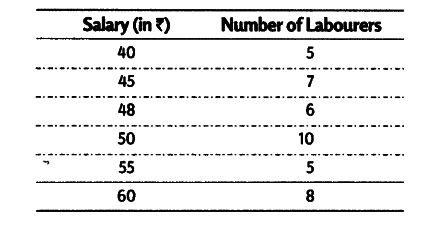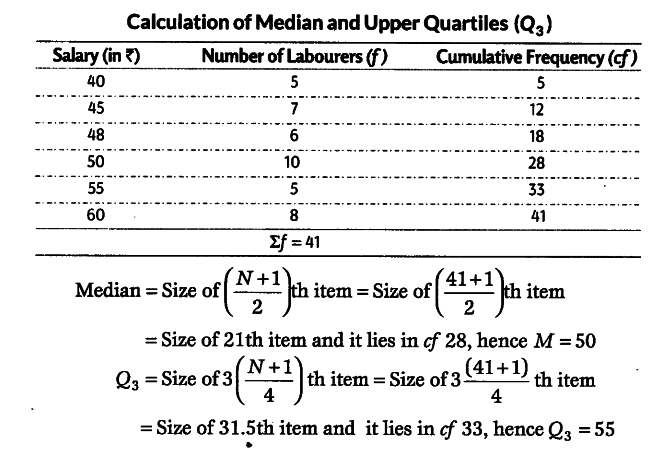# Calculate the median and upper quartile of the following frequency distribution

Calculate the median and upper quartile of the following frequency distribution